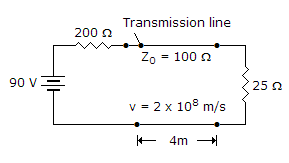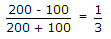# Electronics and Communication Engineering - Microwave Communication - Discussion

### Discussion :: Microwave Communication - Section 1 (Q.No.37)

37.

In the given figure the reflection coefficient at source end is[A]. 1 [B]. -1 [C]. 1/3 [D]. -1/3

Answer: Option C

Explanation:.

 Krishnamachary said: (Sep 30, 2014) As Per The Given Circuit Load Impedance Is 25 Ohms Than Reflection Coefficient Is Modified To (25-100)/(25+100) = (-75)/125 = -0.6.

 Malathi said: (Sep 15, 2016) Look at the question carefully, they mentioned reflection from source end.

 Jagdish said: (May 29, 2017) The reflection coefficient (γ) measures the amplitude of the reflected wave versus the amplitude of the incident wave. The expression for calculating the reflection coefficient is as follows: γ = (ZL - ZS)/(ZL + ZS). Where ZL is the load impedance and ZS is the source impedance. Therefore, (100-200)/(100+200) = -0.33333 i.e. 1/3.

 Saranya said: (May 31, 2017) Yes, your answer was correct @Krishnamachary.

 Sankar said: (Oct 14, 2017) I agree @Malathi, he asked source end so answer 1/3.

 Shubham said: (Feb 7, 2018) It's -1/3. i.e. (-0.333).

#### Post your comments here:

Name *:

Email   : (optional)

» Your comments will be displayed only after manual approval.

#### Current Affairs 2021

Interview Questions and Answers